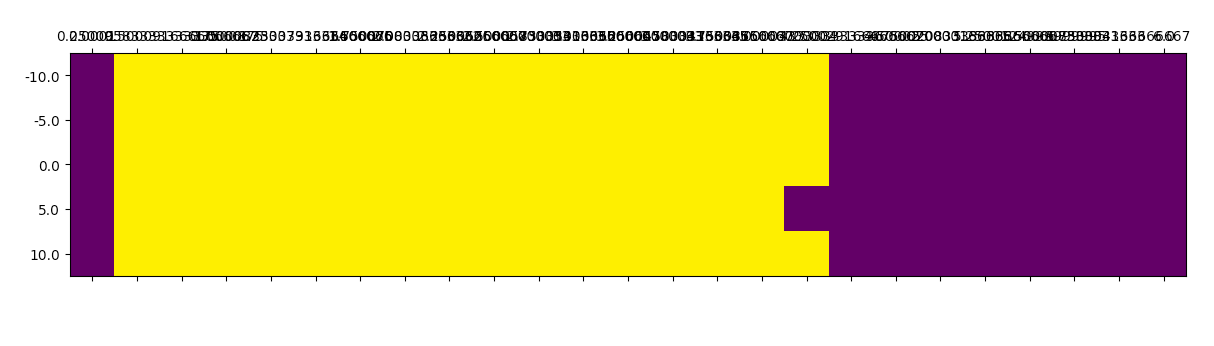# CamDavidsonPilon/lifelines

Have a question about this project? Sign up for a free GitHub account to open an issue and contact its maintainers and the community.

By clicking “Sign up for GitHub”, you agree to our terms of service and privacy statement. We’ll occasionally send you account related emails.

# LogNormalFitter has strange properties #622

Closed
opened this Issue Jan 31, 2019 · 1 comment

Projects
None yet
Owner

### CamDavidsonPilon commented Jan 31, 2019 • edited

 I've spent far too much time trying to debug the LNF model. Trouble is, it's unstable for some inputs. Example: As the following script shows, the model is only stable for ~0.06 < sigma < ~3.5. Anything outside this range will cause the dreaded `Desired error not necessarily achieved due to precision loss` in `minimize`. ``````from lifelines import LogNormalFitter from lifelines.utils import ConvergenceError MU = np.linspace(-10, 10, 5) SIGMA_ = np.linspace(0.0001, 6, 25) R = np.zeros((5, 25)) for i, mu_ in enumerate(MU): for j, sigma_ in enumerate(SIGMA_): try: N = 20000 print(mu_) print(sigma_) X, C = np.exp(sigma_ * np.random.randn(N) + mu_), np.exp(np.random.randn(N) + mu_) E = X <= C T = np.minimum(X, C) LogNormalFitter().fit(T, E) R[i, j] = 1 except ConvergenceError: R[i, j] = 0 plt.matshow(R) plt.xticks(np.arange(25), SIGMA_) plt.yticks(np.arange(5), MU) ``````AFAIK, the log-likelihood and the gradients are computed correctly, though it would be useful to have a second set of eyes on them. Even scipy's `check_gradients` seems to confirm: ``````print(check_grad(_negative_log_likelihood, gradient_function, [0, 0], log(T), E)) `````` When there is no censorship, the model converges for all values... Adding a penalizer doesn't seem to help. When I power transform the durations by the inverse standard deviation of the log(T), this seems to help convergence - however I can't get back the original parameters. Not specifying the gradient function, `jac` seems to help! That is: ``````minimize(_negative_log_likelihood, init, args=(log(T), E), method='BFGS') `````` converges, but ``````minimize(_negative_log_likelihood, init, args=(log(T), E), method='BFGS', jac=gradient_function) `````` seems to fail
Owner Author

### CamDavidsonPilon commented Jan 31, 2019

 I removed the scaling in the exp domain, and this seemed to really help. Only very small values of sigma will cause problems.

Merged

###CamDavidsonPilon closed this in #624 Feb 2, 2019

to join this conversation on GitHub. Already have an account? Sign in to comment
You can’t perform that action at this time.